### Home > CALC > Chapter 6 > Lesson 6.1.3 > Problem6-39

6-39.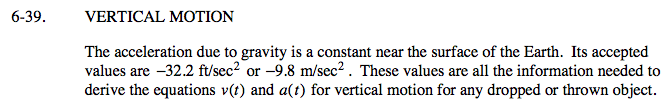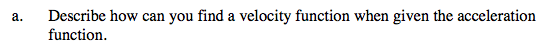Acceleration is the rate of change of velocity...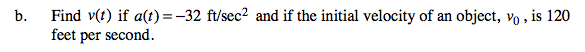There is a relationship between the +C and the initial velocity.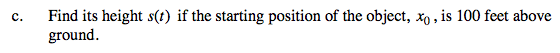Velocity is the rate of change of position...

s(t) = −16t2 + 120t + 100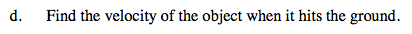First find the TIME when the object hits the ground: s(t) = 0.Then evaluate v(t) at that time.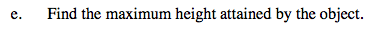Find the vertex of the position function. You could use Algebra or Calculus.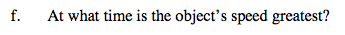Where is the slope of s(t) the steepest? Remember that speed is the absolute value of velocity.